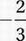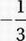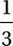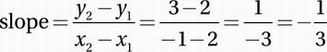# SAT Math Multiple Choice Question 677: Answer and Explanation

### Test Information

Question: 677

2. y = -3(x - 2)2 + 2

In the xy-plane, line l passes through the point (-1, 3) and the vertex of the parabola with equation above. What is the slope of line l?

• A.• B.• C.• D.